# Parallel Invoke in C#

## Parallel Invoke Method in C# with Examples

In this article, I am going to discuss the Parallel Invoke Method in C# with Examples. The Parallel Invoke Method in C# is one of the most frequently used static methods of the Parallel class. So far, we have used a Parallel For Loop and Parallel Foreach Loop to run the same block of code multiple times in parallel. However, sometimes we want to invoke different methods in parallel which are not part of the code block of a loop. For this, we can use the Parallel Invoke method in C#.

##### Example to understand Parallel Invoke Method in C#

The Parallel Invoke method in C# is used to launch multiple tasks that are going to be executed in parallel. Let us first create one example where we will invoke three independent methods sequentially and then we will rewrite the same example where we invoke the same three independent methods parallelly using the Parallel Invoke method. In the below example, we have called Method1, Method2, and Method3 and then log how much time these three methods took the complete the execution in the console window.

```using System;
using System.Diagnostics;

namespace ParallelProgrammingDemo
{
public class Program
{
static void Main()
{
Stopwatch stopWatch = new Stopwatch();

stopWatch.Start();
//Calling Three methods sequentially
Method1();
Method2();
Method3();
stopWatch.Stop();

Console.WriteLine(\$"Sequential Execution Took {stopWatch.ElapsedMilliseconds} Milliseconds");
}
static void Method1()
{
}
static void Method2()
{
}
static void Method3()
{
}
}
}```
###### Output: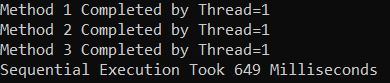As you can see in the above image, all three methods are executed by a single thread and it will take approximately 649 Milliseconds in my machine to complete the execution. Now, we will see the same example using Parallel.Invoke method which will execute these three methods parallelly. What we need to do is, just need to pass the method names to the Parallel.Invoke method as shown in the below image.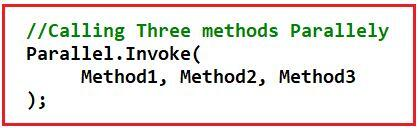The complete example code is given below.

```using System;
using System.Diagnostics;

namespace ParallelProgrammingDemo
{
public class Program
{
static void Main()
{
Stopwatch stopWatch = new Stopwatch();

stopWatch.Start();

//Calling Three methods Parallely
Parallel.Invoke(
Method1, Method2, Method3
);

stopWatch.Stop();
Console.WriteLine(\$"Parallel Execution Took {stopWatch.ElapsedMilliseconds} Milliseconds");

}
static void Method1()
{
}
static void Method2()
{
}
static void Method3()
{
}
}
}
```
###### Output: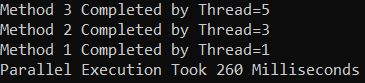As you can see here, the three different methods are executed by three different threads and also it looks 260 Milliseconds on my machine. If you observe it is taking less time than the sequential execution. But this is not always going to be the same i.e. sometimes the sequential execution will take less time than the parallel execution if the task that we are going to perform is very less. So, always it is recommended to do a performance measurement before selecting whether you want to execute methods parallelly or sequentially.

##### Example to Invoke Different Types of Methods using Parallel.Invoke in C#:

The following example demonstrates how to use the Parallel Invoke method in C# with normal methods, anonymous methods (delegates), and lambda expressions.

```using System;

namespace ParallelProgrammingDemo
{
public class Program
{
static void Main()
{
Parallel.Invoke(
NormalAction, // Invoking Normal Method
delegate ()   // Invoking an inline delegate
{
},
() =>   // Invoking a lambda expression
{
}
);
Console.WriteLine("Press any key to exist.");
}
static void NormalAction()
{
}
}
}```
###### Output: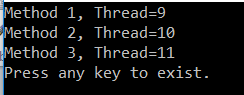The Parallel Invoke method is used to execute a set of operations (actions) in parallel. As you can see in the above output three threads are created to execute three actions which prove that this parallel Invoke Method executes the actions in parallel.

Note: The Parallel Invoke method in C# does not give you any guarantees about the order in which the actions are executed. Each time you execute the code, you may get a different order of output. Another important point that you need to remember is that this method is going to return when all the actions invoked by this method complete their execution.

##### ParallelOptions Class in C#

As we already discussed, using the ParallelOptions class instance, we can limit the number of concurrently executing loop methods. The same thing can also be done with the Invoke method. So, using the Degree of parallelism we can specify the maximum number of threads to be used to execute the program.

##### Example to Understand ParallelOptions Class in C# with Parallel Invoke Method

In the following example, we are creating seven actions without specifying a limit to the number of parallel tasks. So, in this example, it may be possible that all seven actions can be executed concurrently.

As you can see in the below example, we are calling the DoSomeTask method seven times using the Parallel Invoke method. As part of the DoSomeTask method, we are just printing two messages with a 5000 milliseconds pause between them. The messages show when the task started and ended and by which thread so that you will understand the order of execution.

```using System;

namespace ParallelProgrammingDemo
{
public class ParallelInvoke
{
static void Main()
{
Parallel.Invoke(
);
}
{
//Sleep for 5000 milliseconds
}
}
}```

Now run the application and see the output as shown below. The output may vary in your machine.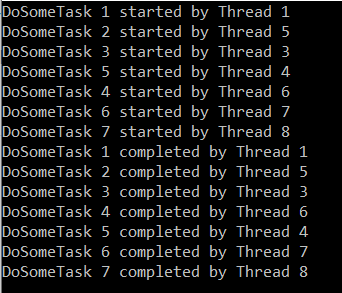You can see in the above output that each of the seven tasks started before any other was completed which proves that all the seven tasks run concurrently. In order to limit the parallelism i.e. to limit the number of threads to execute concurrently, we need to use the ParallelOptions class. We need to pass the object of ParallelOptions to the first parameter of the Invoke method.

##### Example to Limit the Number of Threads to Execute the Methods:

In the below example, we have set MaxDegreeOfParallelism to 3 of ParallelOptions class which will restrict to use of a maximum of three threads to invoke all the methods.

```using System;

namespace ParallelProgrammingDemo
{
public class ParallelInvoke
{
static void Main()
{
//Allowing three task to execute at a time
ParallelOptions parallelOptions = new ParallelOptions
{
MaxDegreeOfParallelism = 3
};
//parallelOptions.MaxDegreeOfParallelism = System.Environment.ProcessorCount - 1;

//Passing ParallelOptions as the first parameter
Parallel.Invoke(
parallelOptions,
);
}
{
//Sleep for 500 milliseconds
}
}
}```
###### Output: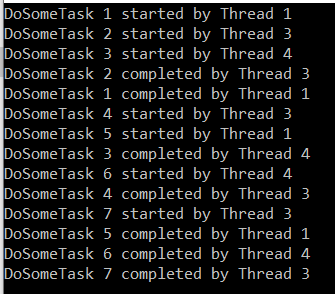As you can see from the above output, the first three tasks have started concurrently as we set the degree of parallelism to 3. When one of the tasks completes its execution then another task started. This process will continue until all of the actions have completed their work. But the most important point that you need to remember is at any given point in time, no more than three tasks are running.

##### Invoking Methods with Input and Return Type using Parallel.Invoke:

As of now, we have discussed the method which does not take any parameter and the return type of those methods is void. Now, let us proceed and try to understand how to invoke methods using Parallel.Invoke with input values and also see how to store the return value of a method. For a better understanding, please have a look at the below example. Here, Method1 does not take any input values but it is returning an integer value. Method2 takes one string value as well as it also returns a string value. And method Method3 takes one integer value and it does not return anything i.e. void.

```using System;

namespace ParallelProgrammingDemo
{
public class Program
{
static void Main()
{
int intResult = 0;
string strResult = string.Empty;
//Calling Three methods Parallely
Parallel.Invoke(
() => intResult = Method1(),
() => strResult = Method2("Pranaya"),
() => Method3(100)
);

Console.WriteLine(\$"Method1 Result: {intResult}");
Console.WriteLine(\$"Method2 Result: {strResult}");
Console.WriteLine(\$"Parallel Execution Completed");

}
static int Method1()
{
return 100;
}
static string Method2(string name)
{
return "Hello:" + name;
}
static void Method3(int number)
{
}
}
}
```
###### Output: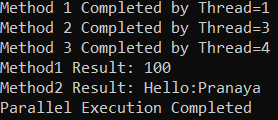In the next article, I am going to discuss How to use the Maximum Degree of Parallelism in C# with Examples. Here, in this article, I try to explain the Parallel Invoke Method in C# with Examples. I hope you understood the need and use of the Parallel Invoke method in C# with Examples.

### 2 thoughts on “Parallel Invoke in C#”

1.Thank you for amazing tutorials!

2.NormalAction, //

Invoking Normal Method in Parallel.Invoke(NormalACtion) not working